## Cubes and Dices

#### Cubes and Dices

1. Choose the cube that will be formed by folding the sheet of paper shown in the problem figure.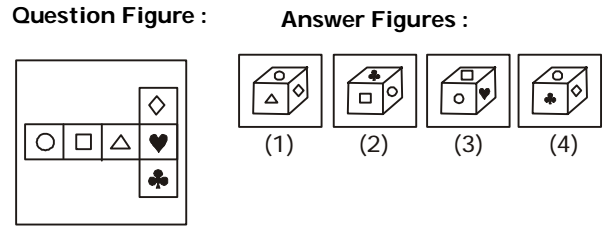1. We follow the given pattern :-
After folding the sheet of paper in the form of a cube:
Ο will lie opposite Δ
□ will lie opposite ♥
◊ will lie opposite ♣
Symbols □ and ♥ cannot be on adjacent faces.
So, Answer Figure (3) can be ruled out.

##### Correct Option: B

We follow the given pattern :-
After folding the sheet of paper in the form of a cube:
Ο will lie opposite Δ
□ will lie opposite ♥
◊ will lie opposite ♣
Symbols □ and ♥ cannot be on adjacent faces.
So, Answer Figure (3) can be ruled out.
Symbols ◊ and ♣ cannot be on adjacent faces.
So, Answer Figure (4) can be ruled out.
Ο and Δ can not be on adjacent sides.
Hence options (1) is not true.
Hence option (2) is true.

1. The solid so formed by joining unit cubes is rotated to obtain different positions, which of these cannot be the shape after it has turned?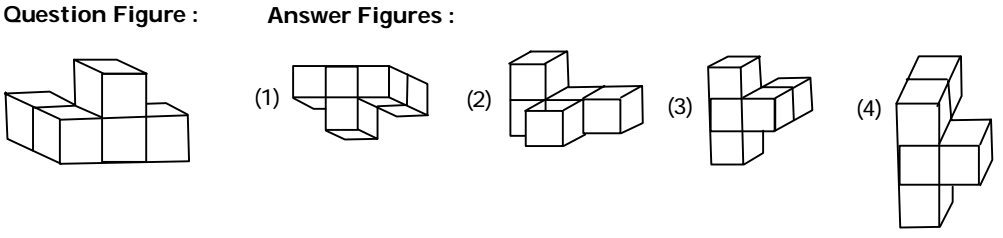1. As per the given pattern in question figure , we can find the similar next position of given dice .
The shape given in the option (3) is not appropriate.

##### Correct Option: C

As per the given pattern in question figure , we can find the similar next position of given dice .
The shape given in the option (3) is not appropriate.

1. Which symbol will appear on the face opposite to the face of a circle O in the cube given below?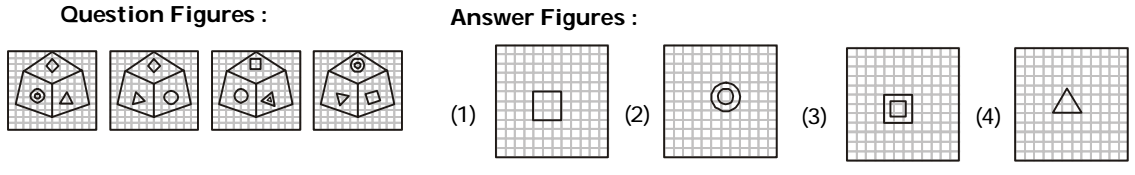1. We follow the given pattern :-
Symbols Δ, ◊, □
and ▲ lie on the faces adjacent to the symbol *Omicron;.

##### Correct Option: B

We follow the given pattern :-
Symbols Δ, ◊, □
and ▲ lie on the faces adjacent to the symbol *Omicron;.
Therefore,◎ lies opposite Ο.

1. How many cubes are there in the diagram?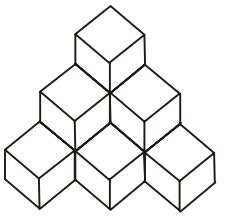1. From the above given diagram , we can say that
Six cubes are visible and four cubes are hidden.

##### Correct Option: A

From the above given diagram , we can say that
Six cubes are visible and four cubes are hidden.
Thus , there are 10 cubes .

1. Six faces of the cube are painted with different colors as shown below. In figure 3, which color is opposite to RED?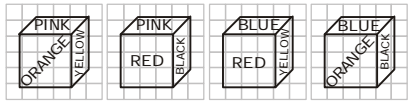1. We follow the given pattern :-
Orange, Yellow, Red and Black colours are on the faces adjacent to Pink colour.

##### Correct Option: C

We follow the given pattern :-
Orange, Yellow, Red and Black colours are on the faces adjacent to Pink colour.
Therefore, Blue colour is opposite to Pink colour.
Pink, Black, Blue and Yellow colours on the faces adjacent to Red colour.
Therefore, Orange colour is opposite to Red colour.